## Behavior of Learning Formula

To understand the behavior of the learning formula , we continue our previous numerical example . Table below show again the user responses together with the failure probability and learning probability at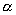= 0.50.

Initially, the learning probability distribution is set as uniform distribution with equal probability of for each character. The learning distribution was then updated using learning formula. Based on the learning distribution, we can design the program response so that the characters that have large learning distribution value will be asked more frequently. In the very long trials, if the user has known all the syllables, the learning distribution should approach uniform distribution again.

## User response

 trial no 0 1 2 3 4 5 6 7 8 9 10 a Wrong Right Wrong Right b Right c Wrong Right Right d Wrong Right

## Probability distribution of failure

 F(a) 0% 30% 31% 20% 21% 24% 26% 26% 27% 25% F(b) 33% 3% 6% 15% 16% 20% 23% 24% 23% 24% F(c) 33% 33% 31% 32% 32% 32% 26% 23% 23% 24% F(d) 33% 33% 33% 33% 32% 24% 26% 26% 26% 27%

## Learning Probability Distribution (= 0.50)

 F(a) 25% 13% 21% 26% 23% 22% 23% 24% 25% 26% 26% F(b) 25% 29% 16% 11% 13% 15% 17% 20% 22% 23% 23% F(c) 25% 29% 31% 31% 31% 31% 32% 29% 26% 25% 24% F(d) 25% 29% 31% 32% 33% 32% 28% 27% 27% 26% 27%

Observe in the table above that the initial probability is very important to determine the behavior of the learning probability distribution. The probability distribution of failure is similar to the learning distribution at zero learning rates. Higher the value of learning rate makes the fluctuation smaller and longer to reach the equilibrium. In this example, the equilibrium values are the initial probabilities (i.e. for each character).

We can view the existing memory in the learning formula dumped the fluctuation of failure distribution. For example, in trial 2 the failure probability of character b drops to 3%, while the learning probability is only drop to 16%. The memory is softening the variability of the distribution. In trial number 4, the user gives correct answer to character a and yield learning probability of 23% for character a and only 13% to character b. Notice that character a now has larger learning probability (will be asked more frequently) than character b because character a has been questioned 2 times and only one answer correct while character b has been asked only one time and it yields correct answer. In trial 7, syllable a, c and d has the same record of one wrong answer and one correct answer. Their learning probabilities, however, are not equal. This is happen because the learning rateis not zero. The learning distribution is updated not only based on the number of correct or wrong answers but also based on the timing to answer it correctly or wrongly. The latest correct answers always get higher learning probability than the old correct answer. At each trial, the learning probabilities are always relative to each other.

Figure below shows the effect of the learning ratetoward the learning probability distribution. We also include time-average for comparison. For this 10 number of trials, the time average is most similar to= 0.623 (by minimizing the absolute different. The value ofis not 0.5 as I first guess it). For longer trials, however, the learning rate will be different by user responses. Sudden change in the distribution is exposed for zero learning rates. For learning rate= 1 , the learning probability distribution is only depending on the initial probability without dynamic update.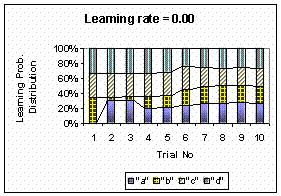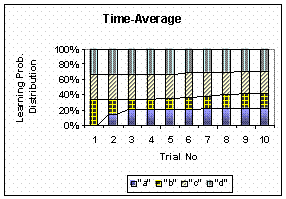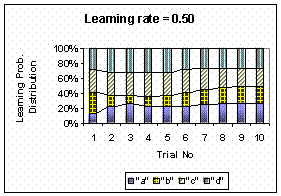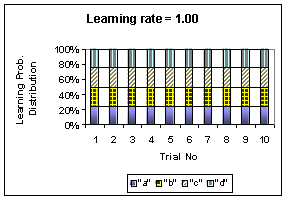It worth to mention that although the updating process is independent among one character to another, the total probability of learning distribution for all characters are always one. It happens because the total probability that character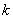has been successfully answered for all characters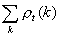is also one. If this total is not guaranteed, the learning probability distribution may get incorrect results.

Preferable reference for this tutorial is

Teknomo, Kardi (2015) Learning Algorithm Tutorials. https:\\people.revoledu.com\kardi\ tutorial\Learning\